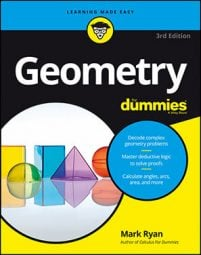##### Geometry For DummiesA translation—probably the simplest type of figure transformation in coordinate geometry—is one in which a figure just slides straight to a new location without any tilting or turning. As you can see, a translation doesn't change a figure's orientation.

It may seem a bit surprising, but instead of sliding a figure to a new location, you can achieve the same end result by reflecting the figure over one line and then over a second line.

You can see how this works by doing the following: Take a blank piece of paper and tear off a little piece of its lower, right-hand corner. Place the sheet of paper in front of you on a desk or table. Now flip the paper to the right over its right edge—you know, so that its right edge doesn't move. You then see the back side of the paper, and the torn-off corner is at the lower, left-hand corner. Finally, flip the paper over to the right again. Now, after the two flips, or two reflections, you see the paper just as it looked originally, except that now it's been slid, or translated, to the right.

Translation line and translation distance: In a translation, the translation line is any line that connects a pre-image point of a figure to its corresponding image point; the translation line shows you the direction of the translation. The translation distance is the distance from any pre-image point to its corresponding image point.

A translation equals two reflections: A translation of a given distance along a translation line is equivalent to two reflections over parallel lines that

• Are perpendicular to the translation line
• Are separated by a distance equal to half the translation distance
Note: The two parallel reflecting lines, l1 and l2, can be located anywhere along the translation line as long as 1) they are separated by half the translation distance and 2) the direction from l1 to l2 is the same as the direction from the pre-image to the image.

Is that theorem a mouthful or what? Instead of puzzling over the theorem, take a look at the next figure to see how reflecting lines work in a translation.After flipping over two reflecting lines, triangle XYZ moves to triangle X'Y'Z'.

Here are a few points about the figure. You can see that the translation distance (the distance from Z to Z') is 20; that the distance between the reflecting lines, l1 and l2, is half of that, or 10; and that reflecting lines l1 and l2 are perpendicular to the translation line, ZZ'.